# 摘要

1952年马柯维茨（Markowitz）在The Journal of Finance（金融学最顶级的学术期刊）上发表了《证券组合选择》论文，开启了现代证券组合管理理论的先河。马柯维茨开创性地引入了均值和方差来定量刻画股票投资的收益和风险（被认为是量化交易策略的鼻祖），建立了确定最佳资产组合的基本模型。其后，越来越多的经济金融学者通过数量化的模型以及周边市场和投资交易问题。夏普（William Sharpe）、林特尔（John Lintner）、特里诺（Jack Treynor）和莫辛（Jan Mossin）分别于1964、1965和1966年在马柯维茨投资组合理论基础上，发展出了资本资产定价模型（CAPM）。该模型不仅提供了评价收益-风险相互转换特征的可运作框架，也为投资组合分析、基金绩效评价提供了重要的理论基础。本文将基于随机森林算法实现多因子的选股策略，既是从N只股票中选择得分最高的前 X%只股票。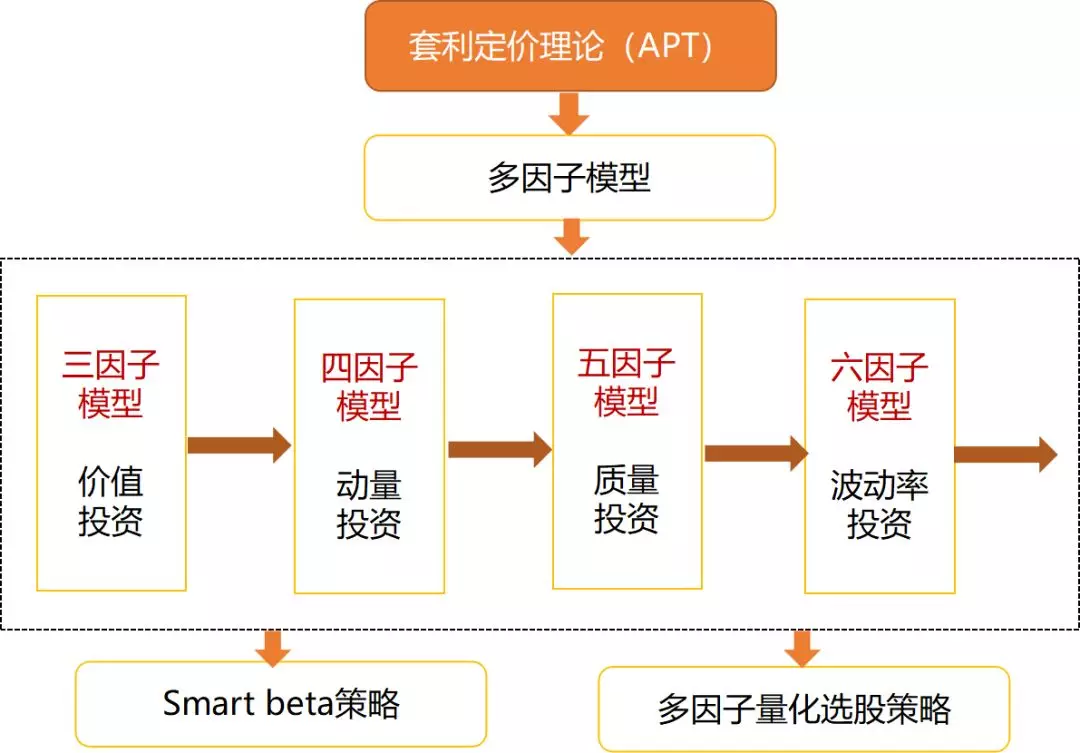# 数据描述

本文选择的数据是从网上爬取的2783家上市公司的股票数据，具体如下图所示，时间段为2012年-2016年，在每年中随机选择一些2到3个样本数据，这样就构成了具有时间序列的股票数据。选择的因子有：,QDturn,QMretnd,QIdxrtn,CR,PB,PS,ROA,ROE,NSR,NQMret；根据上节（https://haosen.blog.csdn.net/article/details/108719768）介绍的构建数据集的方法，构建本样本数据的标签（将股票池中表现好的个股标为类别 1，而表现不好的标类别0等）。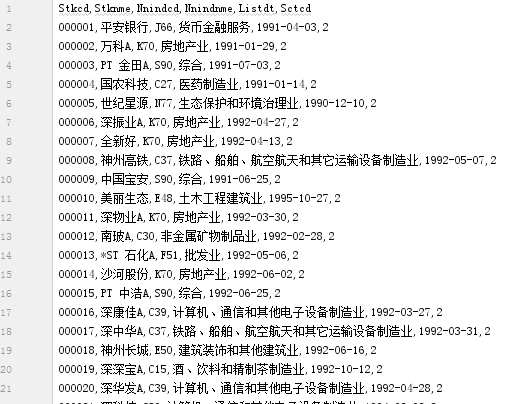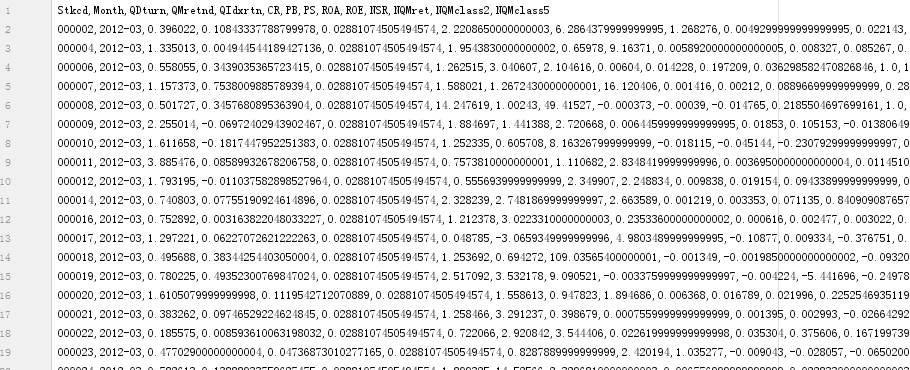for i in range(len(data)-1):
if data['NQMret'].iloc[i]>0:
NQMclass2[i] = 1
elif data['NQMret'].iloc[i]<0:
NQMclass2[i] = 0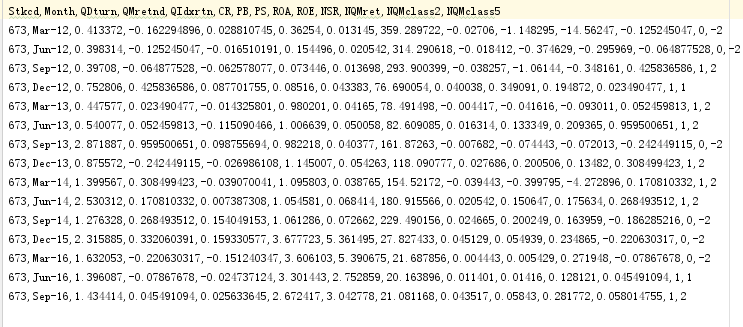# 模型构建

def class2(ntree=20, train=train,test=test):
clf = RandomForestClassifier(n_jobs=2, oob_score=True, n_estimators=ntree)
clf.fit(train[features], train['NQMclass2'])
return(clf)
clf2 = class2(20)
preds = clf2.predict(test[features])
print(pd.crosstab(test['NQMclass2'], preds, rownames=['actual'], colnames=['preds']))
print(clf2.oob_score_)
pd.DataFrame({'features':features,'importance':clf2.feature_importances_}).to_csv('features.csv')  #返回特征值权值
print(clf2.predict(test[features])[:10],clf2.predict_proba(test[features])[:10])

k = []
numb = []
for i in range(30):
numb.append(i+1)
clf2 = class2(i+1)
k.append(clf2.oob_score_)
ntree = pd.DataFrame({'ntree':numb,'oob':k})
ntree.to_csv('ntree.csv')

 def backtestor(self, period, numberofcom):
market = []
ret = []
ret_forecast = []
date = []
timeline =  self.timelist[int(np.where(self.timelist==period)):int(np.where(
self.timelist==period)+1)]
for month in timeline:
r,r_f = backtest.profit(self, month, numberofcom)
m = backtest.marketprofit(self, month)
ret.append(r)
ret_forecast.append(r_f)
market.append(m)
date.append(month)
def cummulti(ret):#累积收益率
cumret = []
c = 1
for i in ret:
c = c*(i+1)
cumret.append(c)
return(cumret)

def yearret(ret):# 年化收益率
# print(ret)
# print(ret.shape)
year_ret = (ret[-1] / ret - 1) / 5
# print(year_ret)
return year_ret

def yearsigma(ret,year_ret):# 夏普比率
ret = np.array(ret)
year_sigma = ret.std() / 5  # 年化收益标准差
# 无风险设为4%
sharpe = (year_ret - 0.04) / year_sigma
return (sharpe)

def Maxdrawdown(ret):# 最大回撤
down_list = []
ret=np.array(ret)
for i in range(1, len(ret)):
down = (1 - ret[i] / ret[:i]).max()
down_list.append(down)
return (max(down_list))


# 结果分析与展示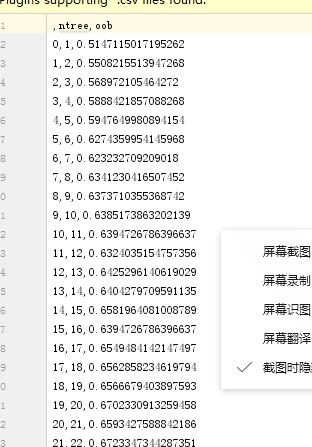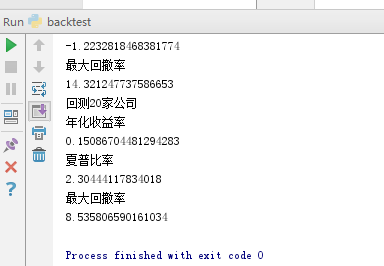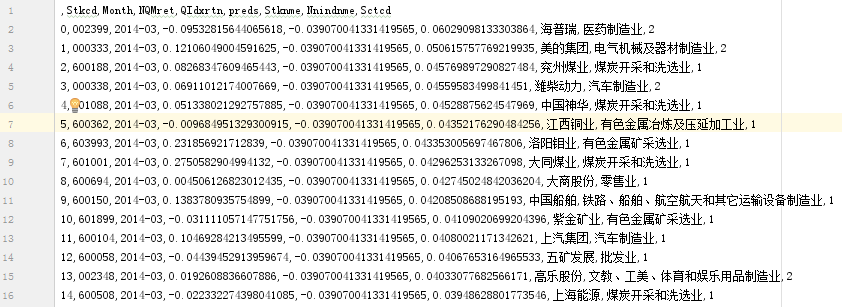# 参考

12-311万+07-253048
06-06578
09-21317
05-2515万+
07-041万+
07-02
04-26